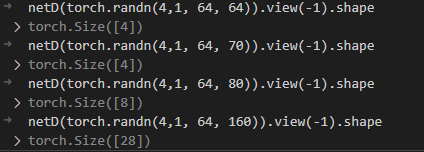# Dimension of output from convolutional network

Hello,

I’m working on implementing a GAN, and have based my GAN on the DCGAN Tutorial here at Pytorch. In my problem the input does not have the same dimensions (which in the tutorial is 64x64), rather I would like it to work with input of dim. 61x250, or something like that. My problem occur when I increase the number of columns in the input image, as the discriminator network then outputs more numbers. I would like the discriminator to output one number per sample in the mini-batch, but for some reason the number of numbers in the output increase as the size of the input image increase.

The code for the discriminator network:
‘’’
class Discriminator(nn.Module):

``````def __init__(self, ngpu, nc, ndf):

super(Discriminator, self).__init__()

self.ngpu = ngpu

self.main = nn.Sequential(

# input is (nc) x 64 x 64

nn.Conv2d(nc, ndf, 4, 2, 1, bias=False),

nn.LeakyReLU(0.2, inplace=True),

# state size. (ndf) x 32 x 32

nn.Conv2d(ndf, ndf * 2, 4, 2, 1, bias=False),

nn.BatchNorm2d(ndf * 2),

nn.LeakyReLU(0.2, inplace=True),

# state size. (ndf*2) x 16 x 16

nn.Conv2d(ndf * 2, ndf * 4, 4, 2, 1, bias=False),

nn.BatchNorm2d(ndf * 4),

nn.LeakyReLU(0.2, inplace=True),

# state size. (ndf*4) x 8 x 8

nn.Conv2d(ndf * 4, ndf * 8, 4, 2, 1, bias=False),

nn.BatchNorm2d(ndf * 8),

nn.LeakyReLU(0.2, inplace=True),

# state size. (ndf*8) x 4 x 4

nn.Conv2d(ndf * 8, 1, 4, 1, 0, bias=False),

nn.Sigmoid()

)

def forward(self, input):

return self.main(input)
``````

‘’’

Screenshot from the VSCode-debugger where I’m illustrating that the dimention of the output increase when I add more columns to the input image.I hope someone are able to see what I’m doing wrong. Thank you!

The `Discriminator` uses a conv layer as it’s output (skip the `nn.Sigmoid` as it’s shape-independent), which makes it usable for different input shapes.
Once way to get a fixed output would be to use e.g. an adaptive pooling layer, which creates a defined spatial activation shape. Based on this comment:

``````# state size. (ndf*8) x 4 x 4
``````

it seems the activation has a spatial shape of `4x4` before being passed to the last conv layer.
Add `nn.Adaptive*Pool2d(output_size=(4, 4))` in front of the last conv layer and it should work (the `*` refers to the pooling modes such as `Avg/Max` etc.).

Thank you, ptrblck, it makes sense and the output of the Discriminator is now as I expect it to be.

I realized now that I have the same problem with the Generator, as it does not output samples of the dimensions I want. Any tips for how to fix the dimensions of the output of this network as well? Now I want it to be 61x250, I can’t see what layers could solve that challenge, and guess this is a more complicated problem.

Generator code:

``````class Generator(nn.Module):
def __init__(self, ngpu,nz,ngf, nc):
super(Generator, self).__init__()
self.ngpu = ngpu
self.main = nn.Sequential(
# input is Z, going into a convolution
nn.ConvTranspose2d( nz, ngf * 8, 4, 1, 0, bias=False),
nn.BatchNorm2d(ngf * 8),
nn.ReLU(True),
# state size. (ngf*8) x 4 x 4
nn.ConvTranspose2d(ngf * 8, ngf * 4, 4, 2, 1, bias=False),
nn.BatchNorm2d(ngf * 4),
nn.ReLU(True),
# state size. (ngf*4) x 8 x 8
nn.ConvTranspose2d( ngf * 4, ngf * 2, 4, 2, 1, bias=False),
nn.BatchNorm2d(ngf * 2),
nn.ReLU(True),
# state size. (ngf*2) x 16 x 16
nn.ConvTranspose2d( ngf * 2, ngf, 4, 2, 1, bias=False),
nn.BatchNorm2d(ngf),
nn.ReLU(True),
# state size. (ngf) x 32 x 32
nn.ConvTranspose2d( ngf, nc, 4, 2, 1, bias=False),
nn.Tanh()
# state size. (nc) x 64 x 64
)
def forward(self, input):
return self.main(input)
``````

The generator might be a bit trickier, since your output shape of odd in one dimension, which doesn’t fit the “doubling” of the spatial size as is currently used (`1x1``4x4``8x8``16x16` → etc.).
To create your desired output shape you would thus need to adapt the transposed conv layers and use their `output_size` argument in their `forward` operation if needed.